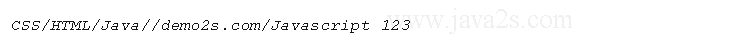# Java array join to String with String separator

## Description

Java array join to String with String separator

```public class Main {
public static void main(String[] argv) throws Exception {
Object[] array = new String[] { "CSS", "HTML", "Java", null, "demo2s.com", "Javascript 123" };
System.out.println(join(array, "/"));
}//www .  jav  a 2s .  c om

/**
* <p>
* Joins the elements of the provided array into a single String containing the
* provided list of elements.
* </p>
*
* <p>
* No delimiter is added before or after the list. A <code>null</code> separator
* is the same as an empty String (""). Null objects or empty strings within the
* array are represented by empty strings.
* </p>
*
* <pre>
* StringUtil.join(null, *)                = null
* StringUtil.join([], *)                  = ""
* StringUtil.join([null], *)              = ""
* StringUtil.join(["a", "b", "c"], "--")  = "a--b--c"
* StringUtil.join(["a", "b", "c"], null)  = "abc"
* StringUtil.join(["a", "b", "c"], "")    = "abc"
* StringUtil.join([null, "", "a"], ',')   = ",,a"
* </pre>
*
* @param array
*          the array of values to join together, may be null
* @param separator
*          the separator character to use, null treated as ""
* @return the joined String, <code>null</code> if null array input
*/
public static String join(Object[] array, String separator) {
if (array == null) {
return null;
}
return join(array, separator, 0, array.length);
}

/**
* <p>
* Joins the elements of the provided array into a single String containing the
* provided list of elements.
* </p>
*
* <p>
* No delimiter is added before or after the list. A <code>null</code> separator
* is the same as an empty String (""). Null objects or empty strings within the
* array are represented by empty strings.
* </p>
*
* <pre>
* StringUtil.join(null, *)                = null
* StringUtil.join([], *)                  = ""
* StringUtil.join([null], *)              = ""
* StringUtil.join(["a", "b", "c"], "--")  = "a--b--c"
* StringUtil.join(["a", "b", "c"], null)  = "abc"
* StringUtil.join(["a", "b", "c"], "")    = "abc"
* StringUtil.join([null, "", "a"], ',')   = ",,a"
* </pre>
*
* @param array
*          the array of values to join together, may be null
* @param separator
*          the separator character to use, null treated as ""
* @param startIndex
*          the first index to start joining from. It is an error to pass in an
*          end index past the end of the array
* @param endIndex
*          the index to stop joining from (exclusive). It is an error to pass
*          in an end index past the end of the array
* @return the joined String, <code>null</code> if null array input
*/
public static String join(Object[] array, String separator, int startIndex, int endIndex) {
if (array == null) {
return null;
}
if (separator == null) {
separator = EMPTY;
}

// endIndex - startIndex > 0: Len = NofStrings *(len(firstString) +
// len(separator))
// (Assuming that all Strings are roughly equally long)
int bufSize = (endIndex - startIndex);
if (bufSize <= 0) {
return EMPTY;
}

bufSize *= ((array[startIndex] == null ? 16 : array[startIndex].toString().length()) + separator.length());

StringBuffer buf = new StringBuffer(bufSize);

for (int i = startIndex; i < endIndex; i++) {
if (i > startIndex) {
buf.append(separator);
}
if (array[i] != null) {
buf.append(array[i]);
}
}
return buf.toString();
}

public static final String EMPTY = "";
}

/*
* you may not use this file except in compliance with the License.
* You may obtain a copy of the License at
*